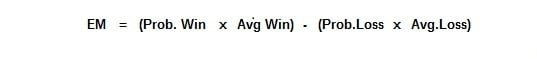# How to calculate the mathematical expectation of a trading system

Calculating the mathematical expectation of a trading system is one of the first things that should be done to know if the system is capable of making money in the long term. Having a positive mathematical expectation is an indispensable condition that any moderately reliable system must meet.

The mathematical expectation measures the amount that is expected to be won or lost on average for each trade we do. For its calculation to be reliable, it is best that we take into account as many trades as possible.

## Calculation of the mathematical expectation of a trading system

The mathematical expectation Ε (X) is defined as “the sum of the probability of each possible event multiplied by the frequency of said process”, and this translated into trading terms is: how much we earn on average in winning trades * the % of success of our system (winning trades) + average loss in losing trades * % of system failures (losing trades).

The formula to calculate the mathematical expectation of the trading system is:where:

• Win Prob = percentage of positive trades
• Avg Win = average profit
• Prob. Loss = percentage of negative trades
• Avg.Loss = average losses

The mathematical expectation tells us the average dollar amount of profit or loss that can be expected in the long term if all available trades are included. Or what is the same: the net profit/total number of trades.

## Conclusions

• To make money with a trading system, it is not necessary to win on all trades, it is the combination of the number of times we win or loss and how much we earn per trade that really counts.
• For a system to be profitable, it must have a positive mathematical expectation. Now, a positive mathematical expectation tells us nothing about the volatility of the results or the winning or losing streaks of a system.